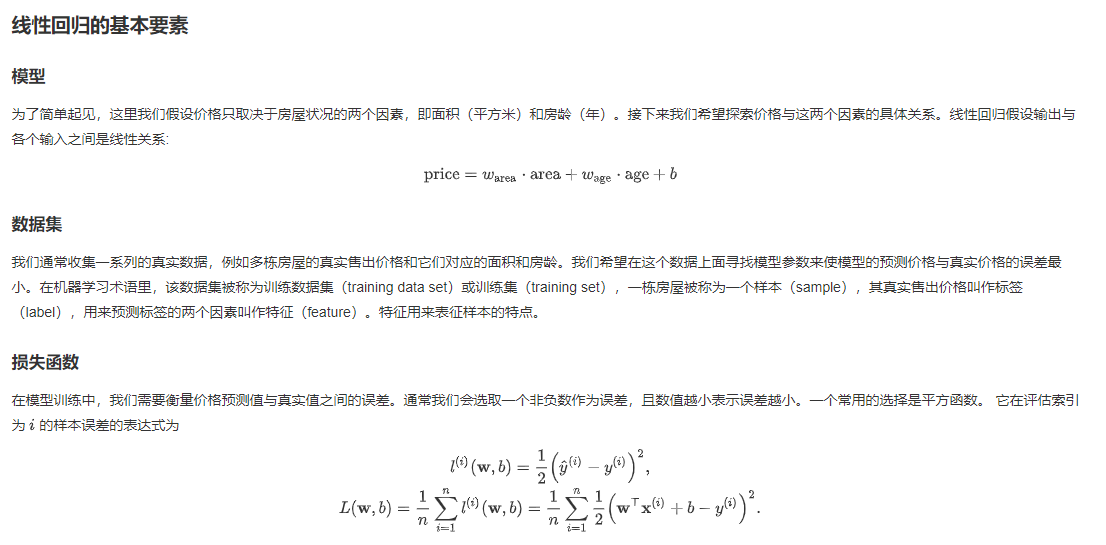## 优化函数 - 随机梯度下降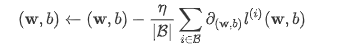def data_iter(batch_size, features, labels):
num_examples = len(features)
indices = list(range(num_examples))
random.shuffle(indices)  # random read 10 samples
for i in range(0, num_examples, batch_size):
j = torch.LongTensor(indices[i: min(i + batch_size, num_examples)]) # the last time may be not enough for a whole batch
yield  features.index_select(0, j), labels.index_select(0, j)


In : num_examples = 20

In : indices = list(range(num_examples))

In : indices
Out: [0, 1, 2, 3, 4, 5, 6, 7, 8, 9, 10, 11, 12, 13, 14, 15, 16, 17, 18, 19]

In : random.shuffle(indices)

In : import random

In : random.shuffle(indices)

In : indices
Out: [8, 16, 15, 18, 14, 10, 0, 7, 2, 3, 4, 9, 12, 6, 17, 13, 5, 11, 19, 1]

In : batch_size = 4

In : for i in range(0, num_examples, batch_size):
...:     print(i)
...:
0
4
8
12
16

• 问题：随便选了课程代码中的几个小细节，考考大家，有没有人来回答一下~
1. torch.mm 和 torch.mul 的区别？
2. torch.manual_seed(1)的作用？
• 答：
(1)torch.mm是矩阵相乘，torch.mul是按元素相乘
(2)设置随机种子，使实验结果可以复现
(3)使梯度置零，防止不同batch得到的梯度累加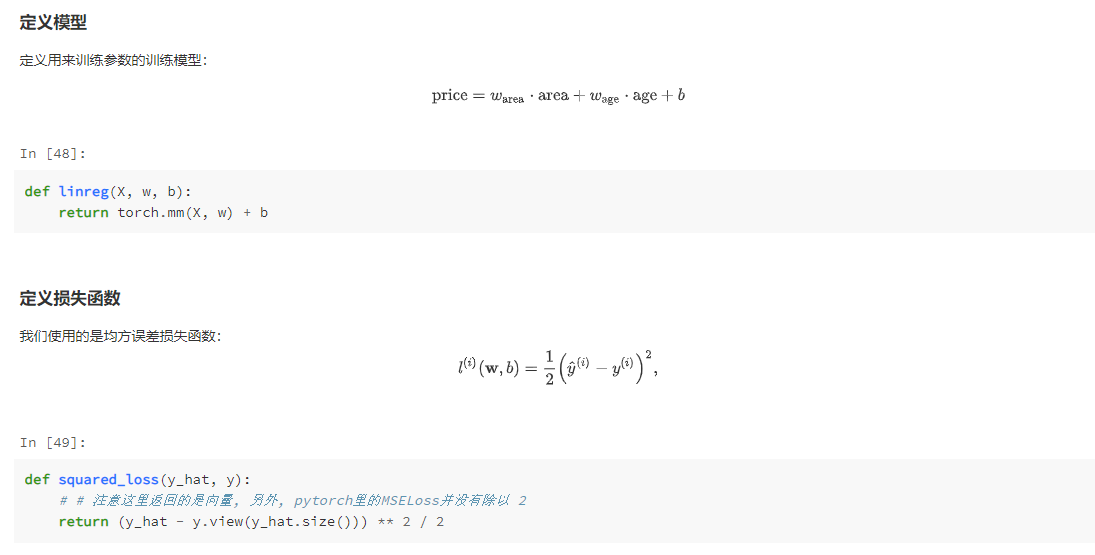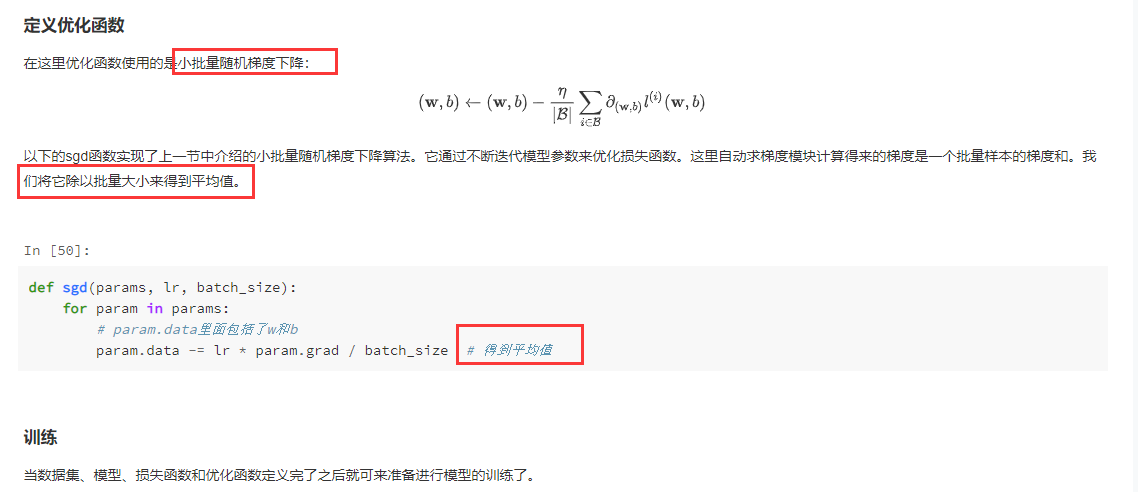## 课后习题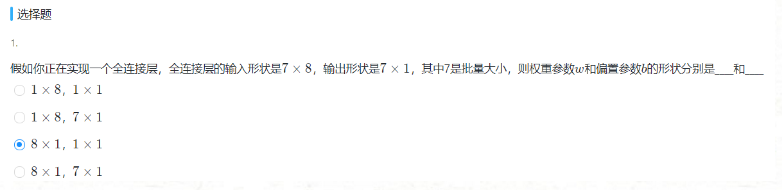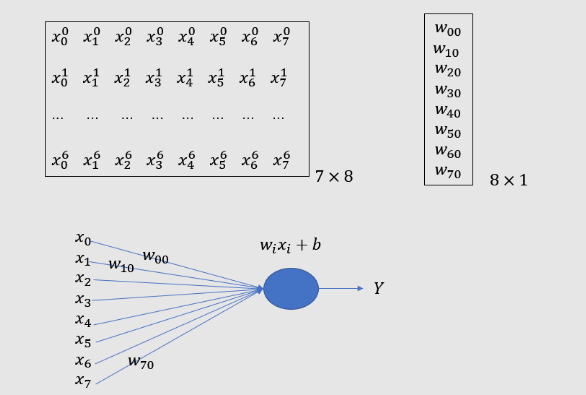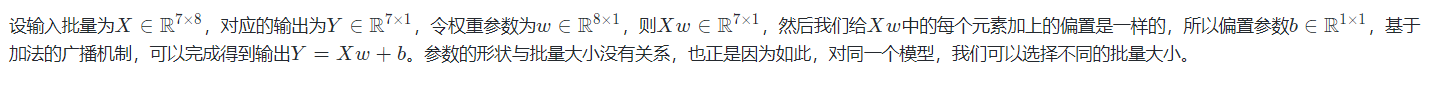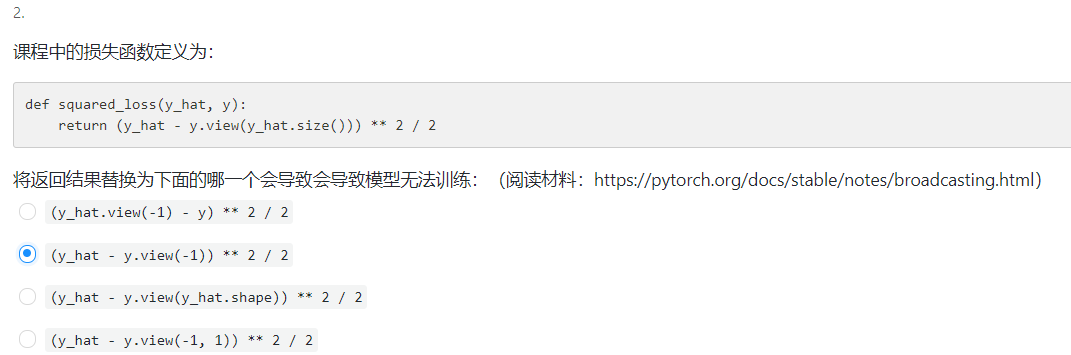In : import numpy as np

In : y = np.array([1,2,3,4,5,7,8])

In : y.shape
Out: (7,)

In : import torch

In : y = torch.Tensor(y)

In : y.view(-1)
Out: tensor([1., 2., 3., 4., 5., 7., 8.])

In : y.view((-1,1))
Out:
tensor([[1.],
[2.],
[3.],
[4.],
[5.],
[7.],
[8.]])

In : y.view((7,1))
Out:
tensor([[1.],
[2.],
[3.],
[4.],
[5.],
[7.],
[8.]])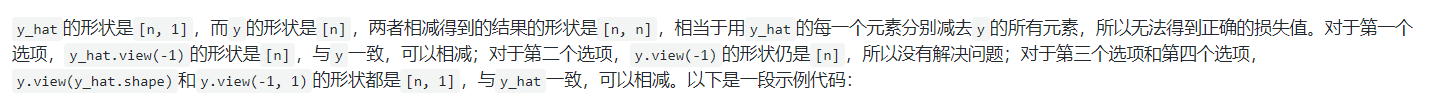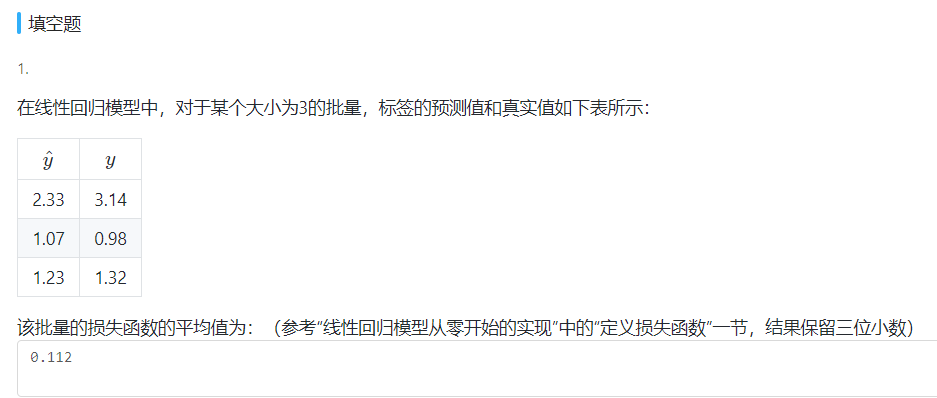In : 0.5* (1/3)*((2.33-3.14)**2 + (1.07 - 0.98)**2 + (1.23 - 1.32)**2)
Out: 0.11205000000000001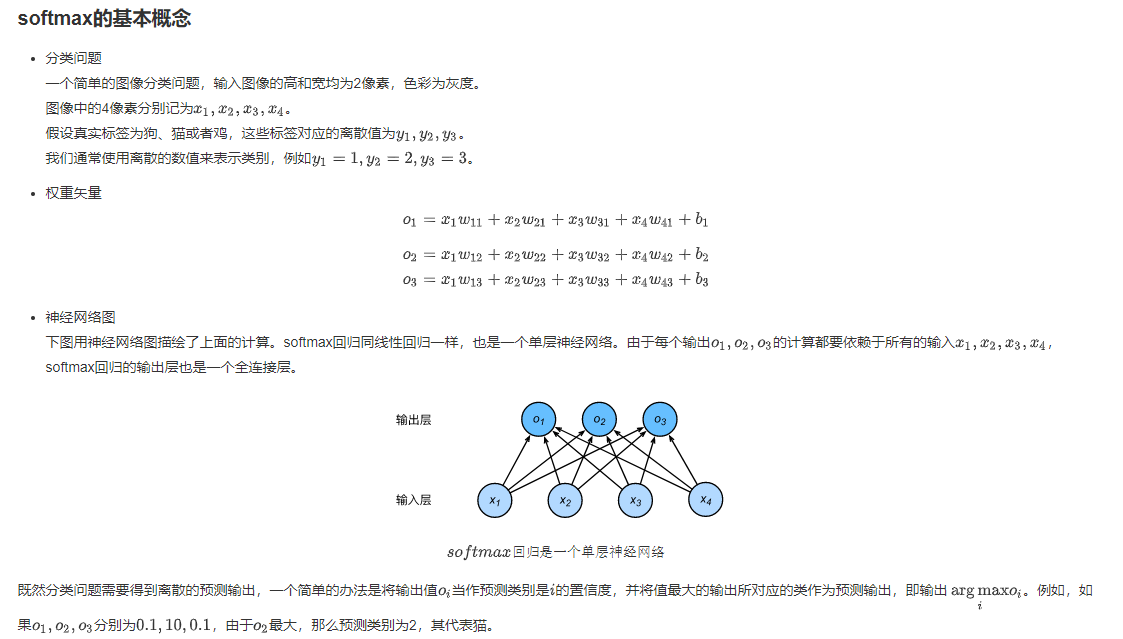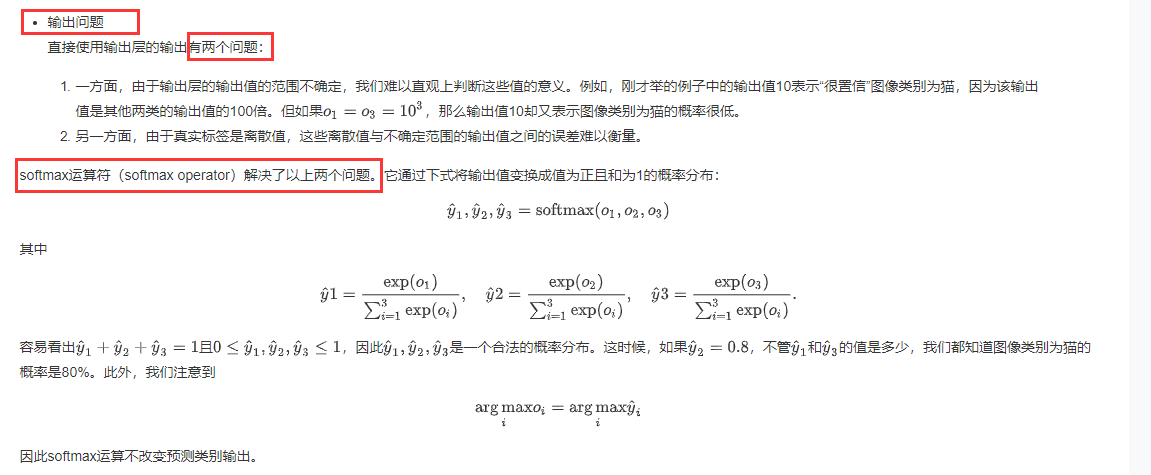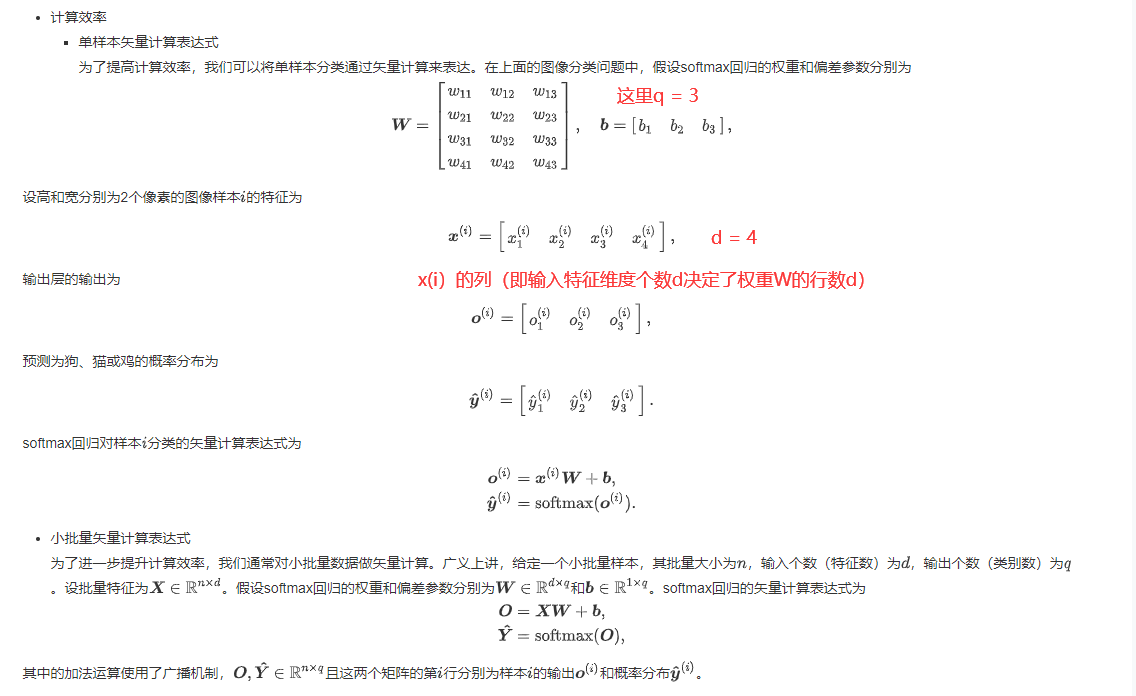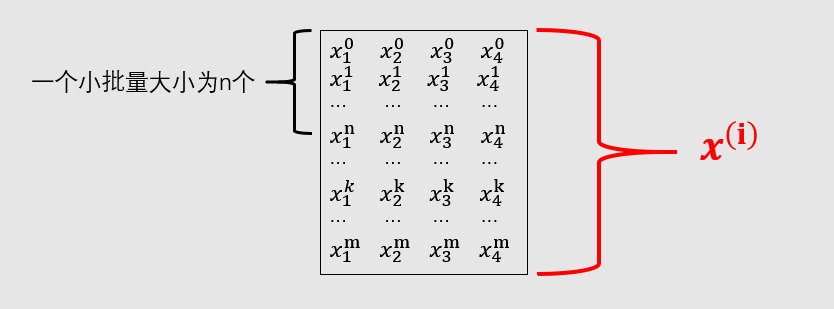• 这里特征个数d = 4, q = 3（对应输出o(i)的个数或者截距b(i)的个数）， W是4×3型，b是1×3型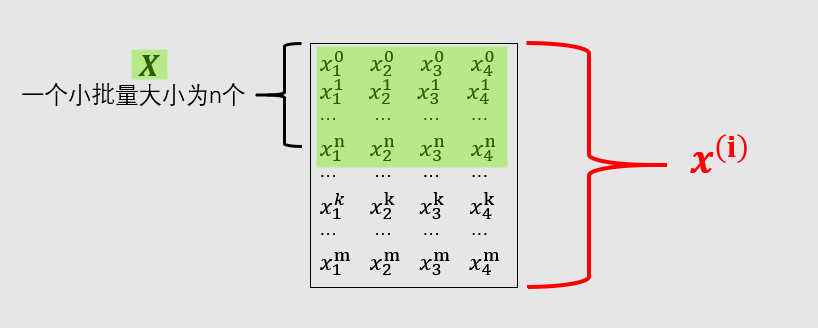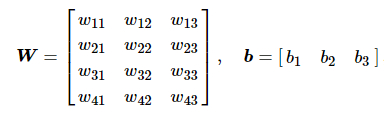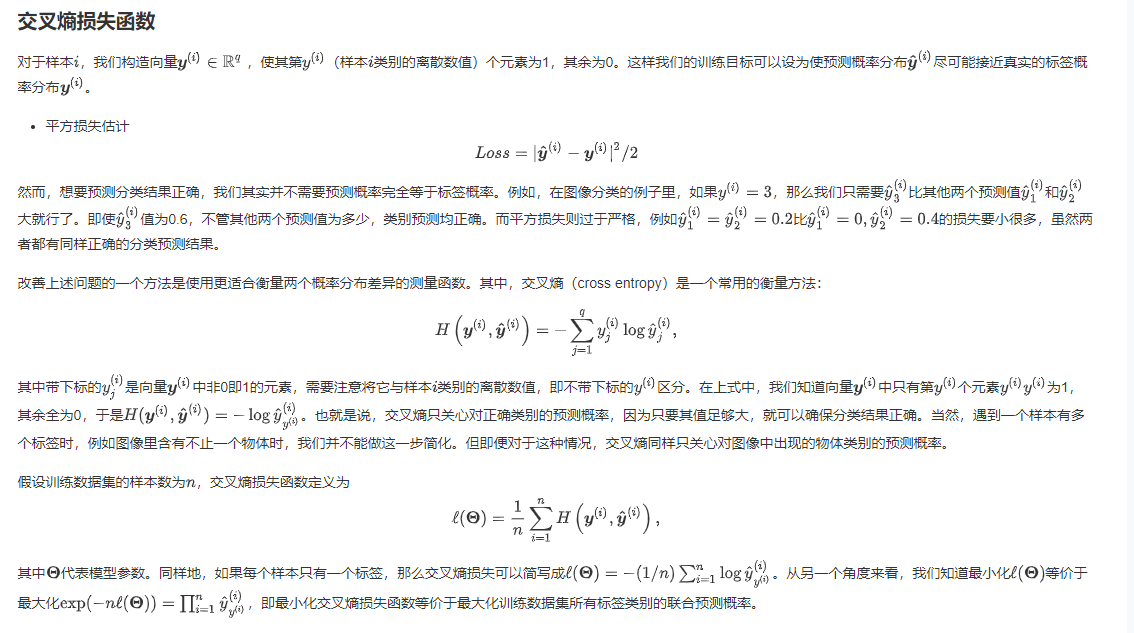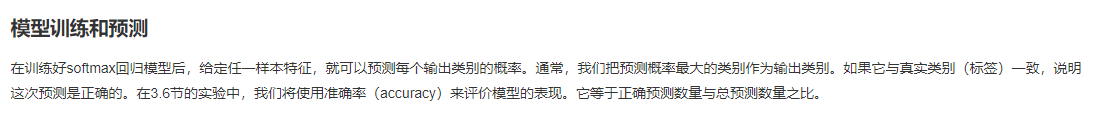• 交叉熵损失函数原理详解

https://blog.csdn.net/b1055077005/article/details/100152102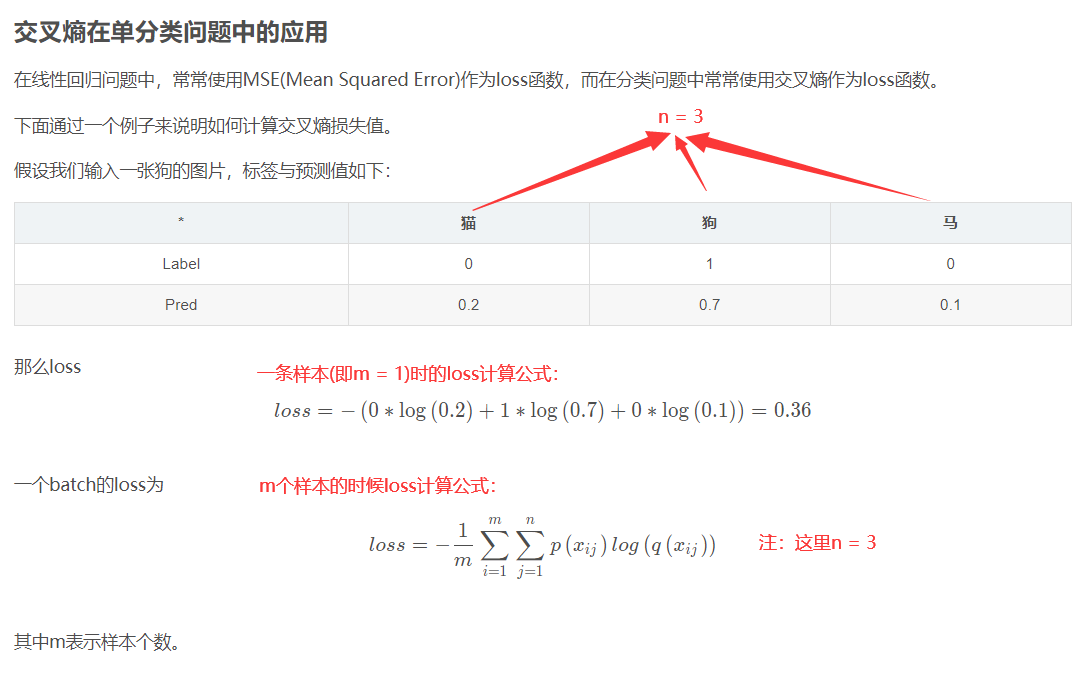• 代码片段解读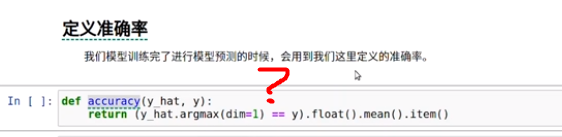def accuracy(y_hat, y):
return (y_hat.argmax(dim=1) == y).float().mean().item()

• 代码分析
In : y_hat = torch.tensor([[0.1, 0.3, 0.6], [0.3, 0.2, 0.5]])^M
...: y = torch.LongTensor([0, 2])   # 真实的标签值

In : y.view(-1, 1)
Out:
tensor([,
])

In : y_hat.gather(1, y.view(-1, 1))
Out:
tensor([[0.1000],
[0.5000]])

In : y_hat.argmax(dim=1)
Out: tensor([2, 2])

In : (y_hat.argmax(dim=1) == y)
Out: tensor([0, 1], dtype=torch.uint8)  # 第一个预测错误，所以值为0，第二个预测正确，所以值为1

In : (y_hat.argmax(dim=1) == y).float()
Out: tensor([0., 1.])

In : (y_hat.argmax(dim=1) == y).float().mean()
Out: tensor(0.5000)

In : (y_hat.argmax(dim=1) == y).float().mean().item()
Out: 0.5



2个样本里面只对了一个样本（即最后一个），第一个真实值为标签0，但是y_hat将其预测为标签2。

### 总结

softmax的常数不变性引发的思考：

SoftMax回归：预测离散值，用于分类，损失函数通常用交叉熵，softMax这一步主要用于归一化，得到概率分布
SoftMax对输出层进行归一化的原因:
1、输出层的输出值的范围不确定，难以直观上判断这些值的意义。
2、由于真实标签是离散值，这些离散值与不确定范围的输出值之间的误差难以衡量。

## 课后习题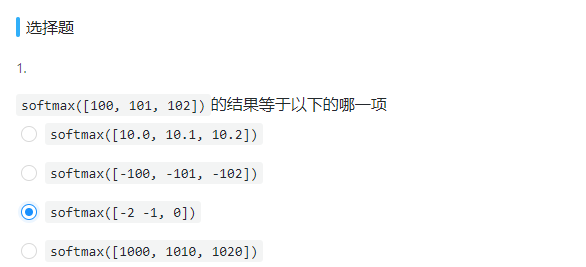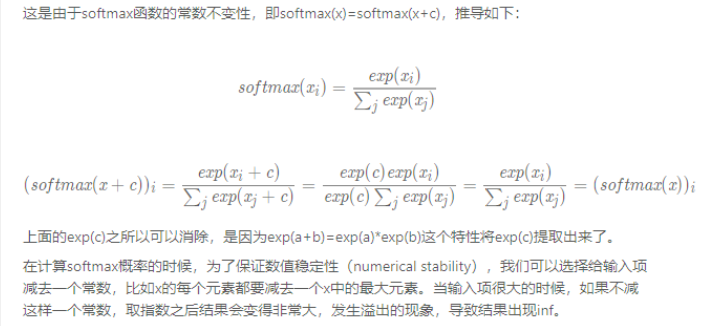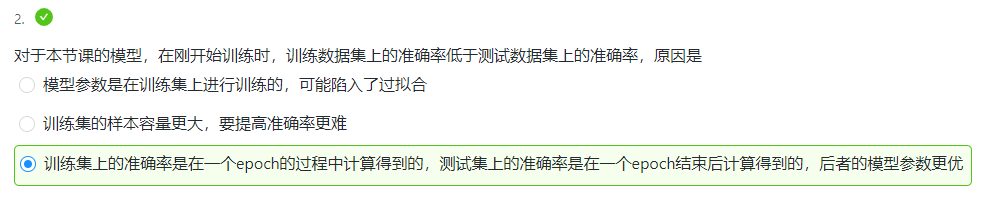• softmax知识点回顾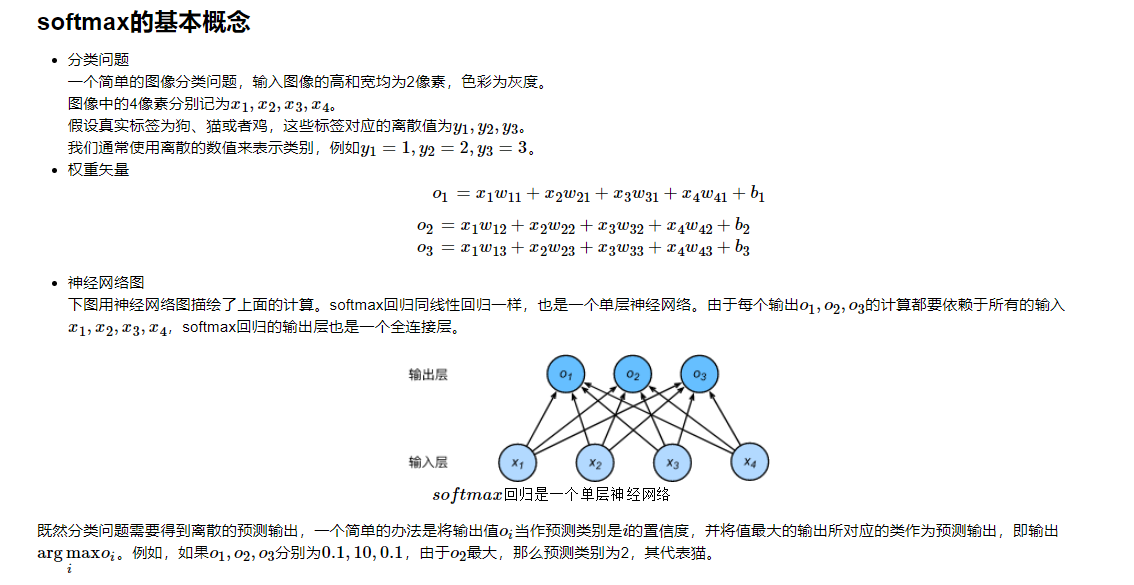• 多层感知机基本知识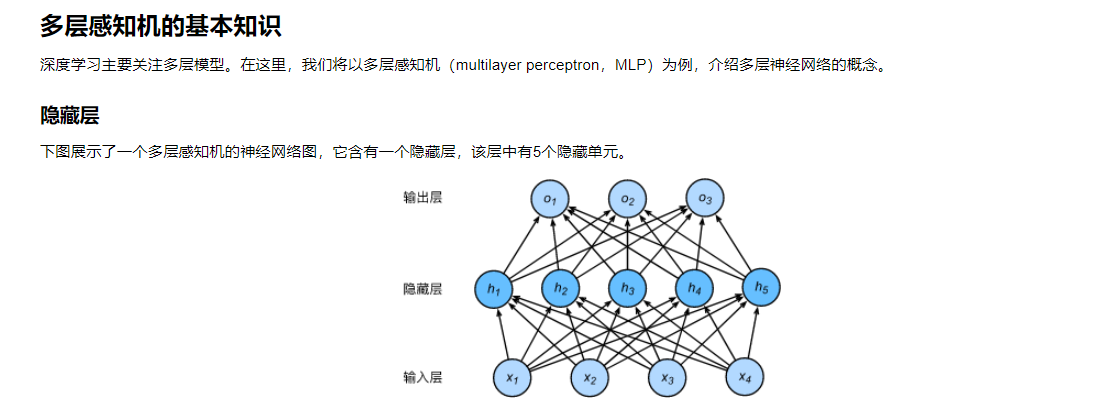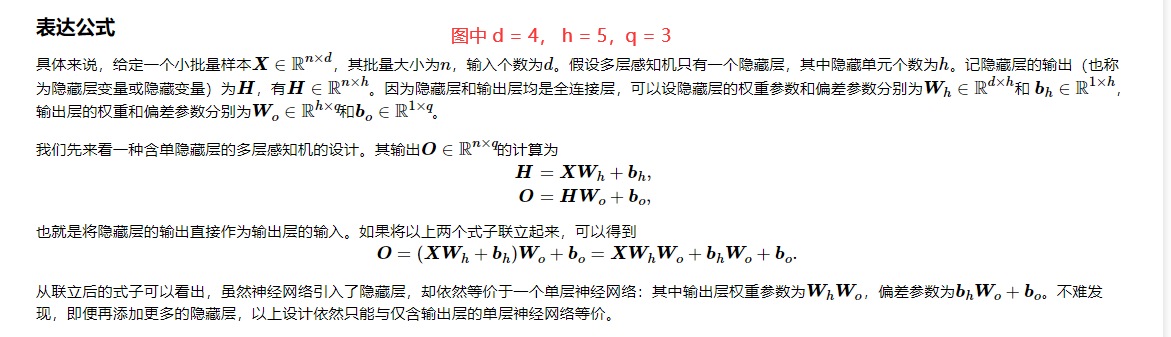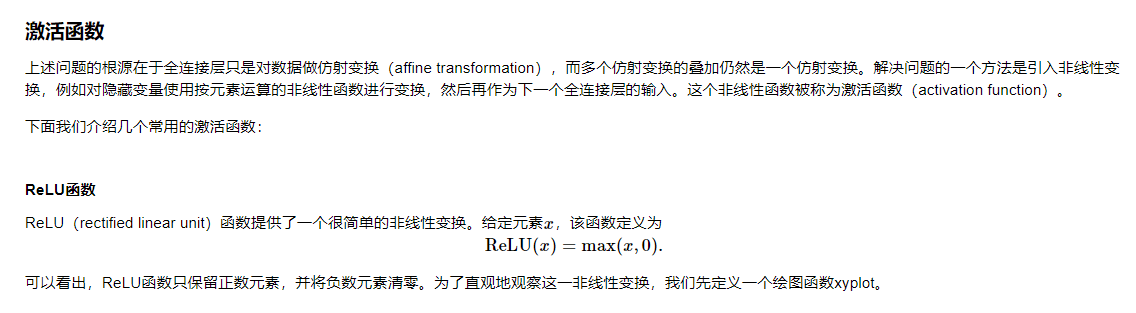• 关于激活函数的选择

ReLu函数是一个通用的激活函数，目前在大多数情况下使用。但是，ReLU函数只能在隐藏层中使用。

## 课后习题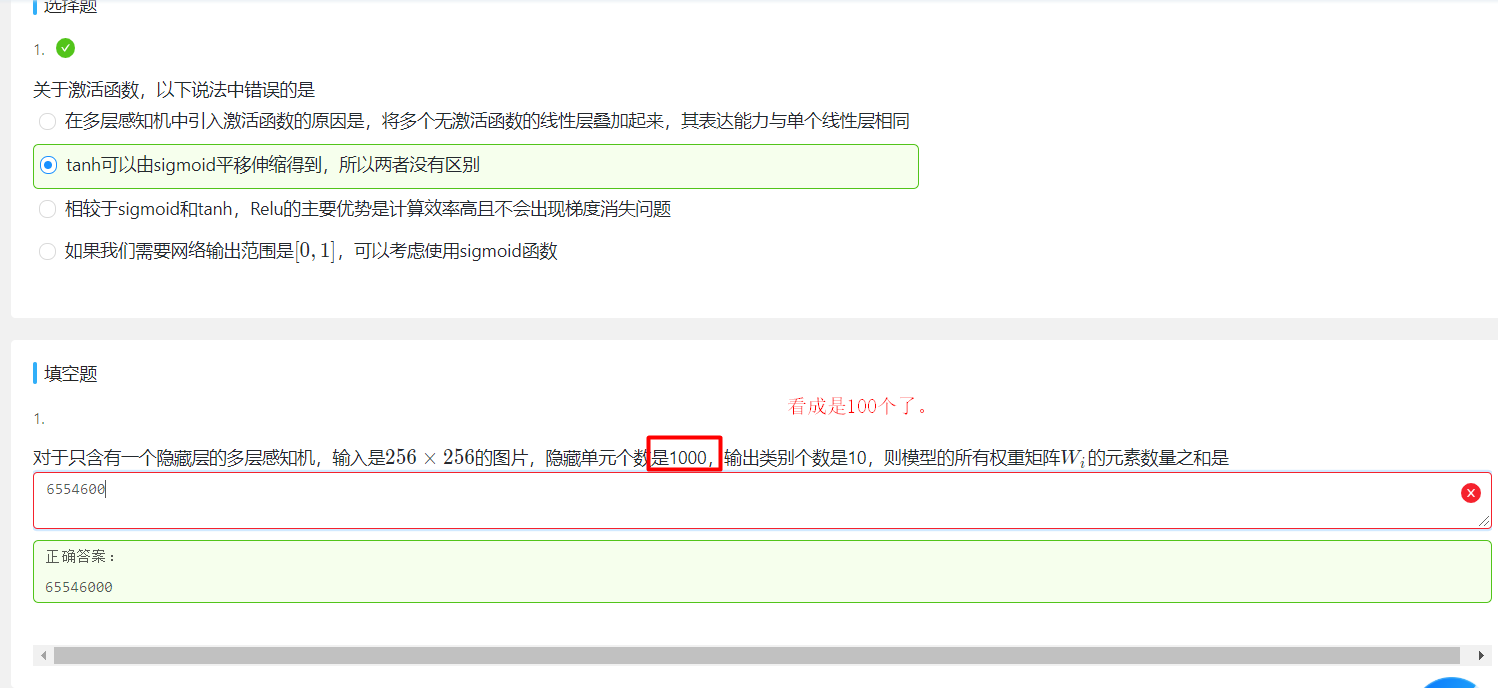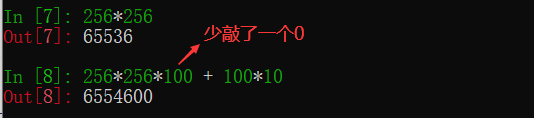• 知识点补充

(1). PyTorch 的 backward 为什么有一个 grad_variables 参数？ - Towser的文章 - 知乎
https://zhuanlan.zhihu.com/p/29923090

(2). 详解Pytorch 自动微分里的（vector-Jacobian product） - mathmad的文章 - 知乎 【推荐看看】
https://zhuanlan.zhihu.com/p/65609544

posted on 2020-02-14 20:55  星辰之衍  阅读(395)  评论(0编辑  收藏